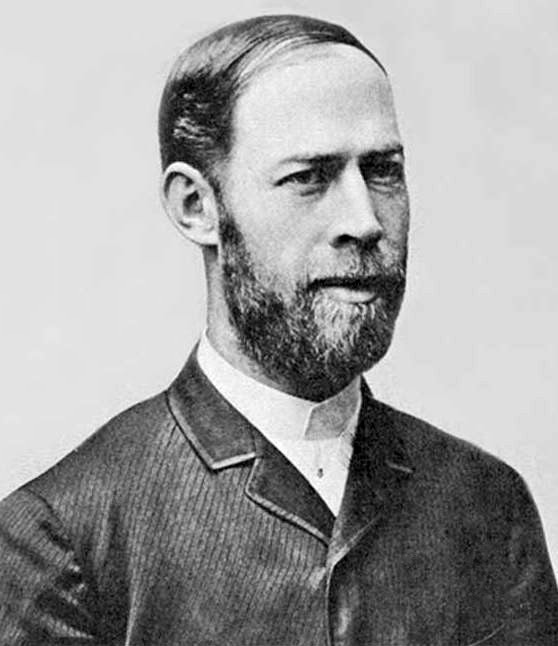### FCC Technician Exam Question Of The Day (T5C05)

Q) What is the unit of frequency?

A) Hertz

NJ2X Notes:
The unit of measure for frequency (hertz) is named after named after the German physicist Heinrich Hertz for his work in the electromagnetic theory of light.  The SI unit "hertz" is abreviated as "Hz".  Frequency in hertz is the number of cycles of a repeating event per unit time measured in seconds.  So 1Hz = 1 cycle per second. ﻿Heinrich Hertz